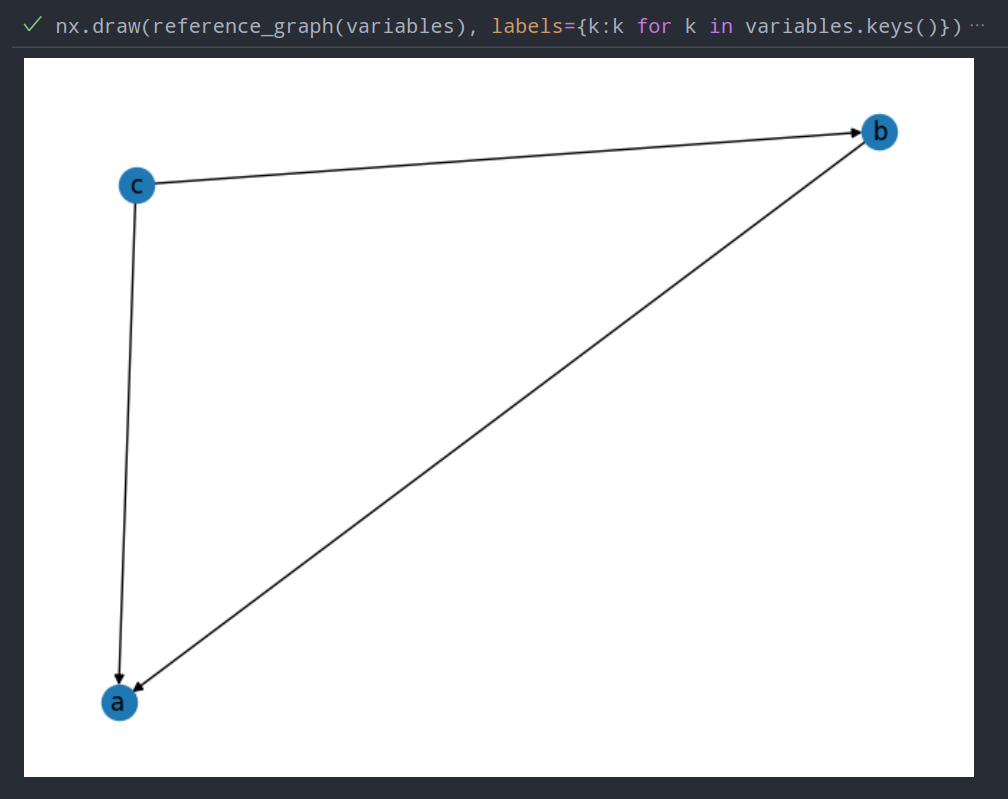### Debugging python memory leaks

Some notes so I don't forget

Quick notes:

• You can get the size of an object using sys.getsizeof, see this SO answer for more depth.
• You can get memory usage of the python process with process = psutil.Process(os.getpid()) then bytes_used = process.memory_info().rss, showing diffs after calling suspicious functions can be good
• The garbage collector interface is powerful. Take a quick look at pydoc gc, in particular:
• get_objects() will give you a list of all objects currently tracked by the collector
• get_referrers() and get_referents() allow you to walk the graph of which objects have references to each other

Here's an example of what you can do with this knowledge and a little imagination. Note the following function isn't complete, you'd want to make it recursive and restrict the depth somehow.

import matplotlib.pyplot as plt
import networkx as nx
import gc

# NOTE: Unfinished, will maybe finish tomorrow
def reference_graph(variables: dict):
ids = {id(o): name for name, o in variables.items()}
def _name(o):
return ids[id(o)]

G = nx.DiGraph()
for o1 in variables.values():
for o2 in (o2 for o2 in gc.get_referents(o1) if id(o2) in ids):
for o2 in (o2 for o2 in gc.get_referrers(o1) if id(o2) in ids):
return G

# Test it
a = ['foo', 'bar']
b = [[a], 'baz']
c = ['qux', b]

variables = {'a': a, 'b': b, 'c': c}
nx.draw(reference_graph(variables), labels={k:k for k in variables.keys()})
plt.show()

Here's the graph from my messy version that works slightly betterThis is probably not super helpful, but it shows an interesting trick.